You are currently offline. Some features of the site may not work correctly.

# (ε, δ)-definition of limit

Known as: Epsilontic, Delta epsilon proof, Epsilon, delta method
In calculus, the (ε, δ)-definition of limit ("epsilon-delta definition of limit") is a formalization of the notion of limit. The concept is due to… Expand
Wikipedia

## Papers overview

Semantic Scholar uses AI to extract papers important to this topic.
2011
2011
• 2011
• Corpus ID: 45669218
This paper investigates some initial value problems in discrete fractional calculus. We introduce a linear difference equation of… Expand
•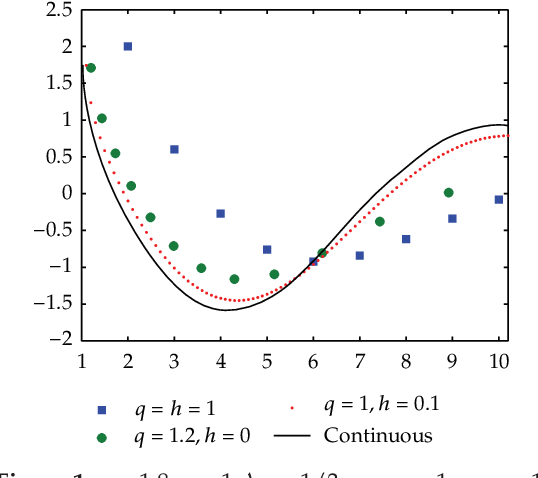Is this relevant?
2010
2010
• 2010
• Corpus ID: 3685625
In this paper, a multiple-source-point boundary-collocation Trefftz method, with characteristic lengths being introduced in the… Expand
••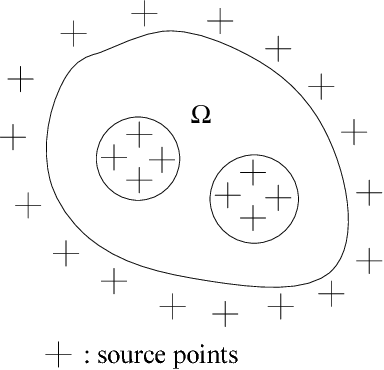•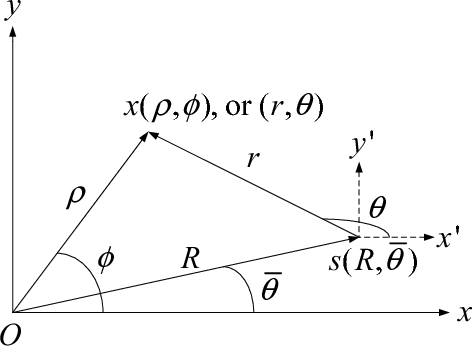•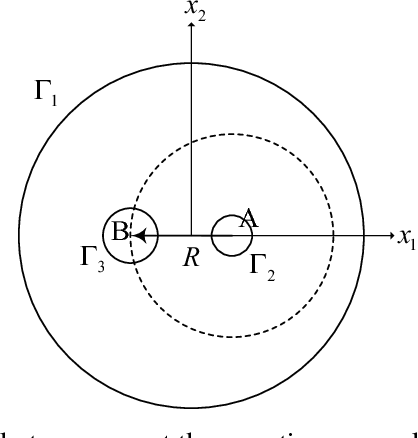•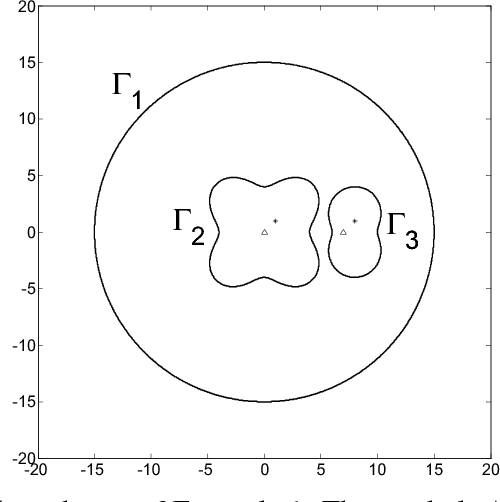Is this relevant?
Highly Cited
2010
Highly Cited
2010
• POPL '10
• 2010
• Corpus ID: 795130
We present an analysis to automatically determine if a program represents a continuous function, or equivalently, if… Expand
•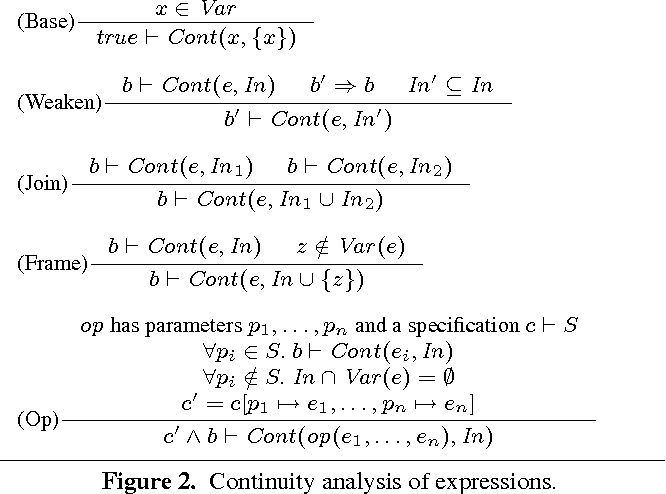•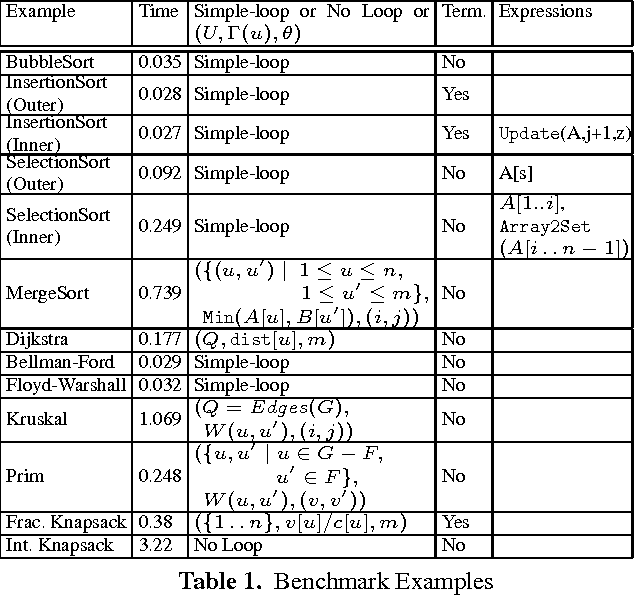•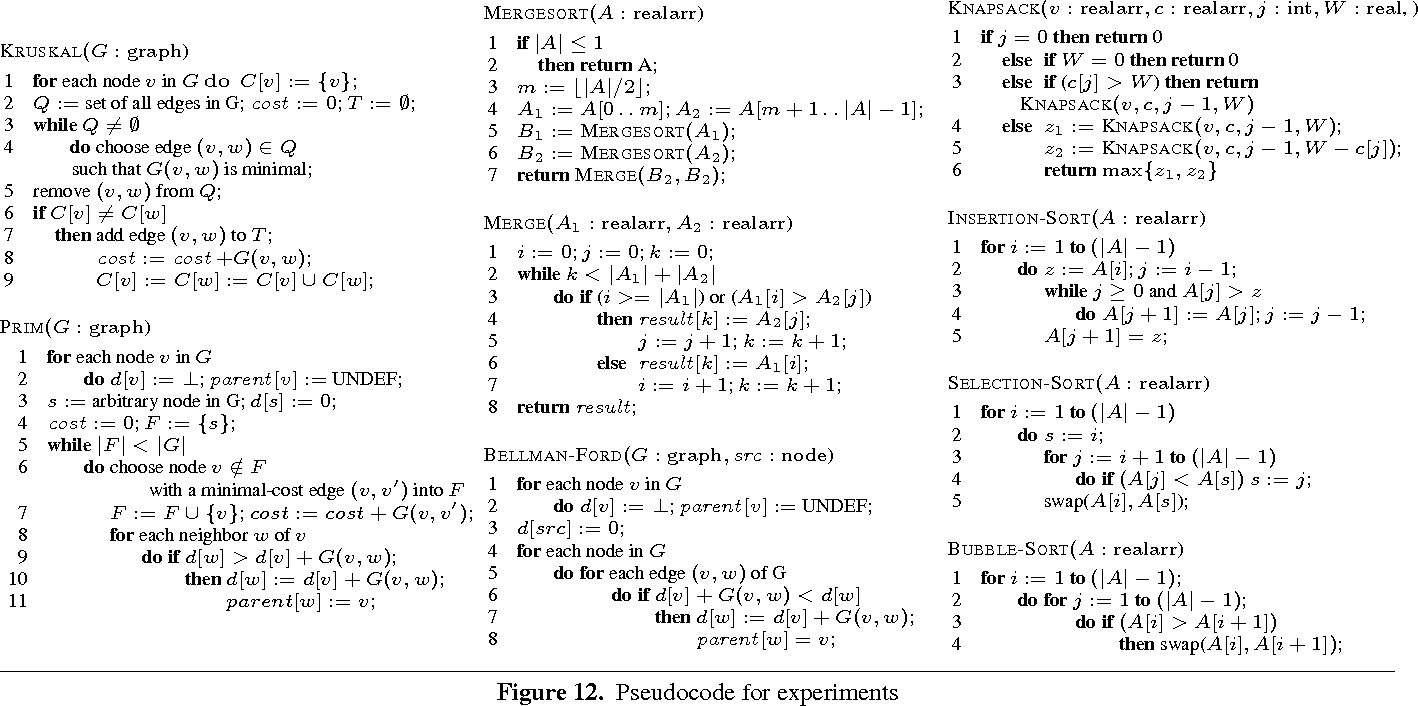Is this relevant?
Highly Cited
2009
Highly Cited
2009
• 50th Annual IEEE Symposium on Foundations of…
• 2009
• Corpus ID: 8524267
For arbitrarily small constants epsilon, delta ≫ 0\$, we present a long code test with one free bit, completeness 1-epsilon and… Expand
Is this relevant?
2008
2008
• Appl. Math. Lett.
• 2008
• Corpus ID: 21120263
In this work we consider a Cauchy problem for a nonlinear viscoelastic equation. Under suitable conditions on the initial data… Expand
Is this relevant?
2008
2008
Imagine asking a first-semester calculus student to explain the definition of the derivative using the epsilon-delta definition… Expand
•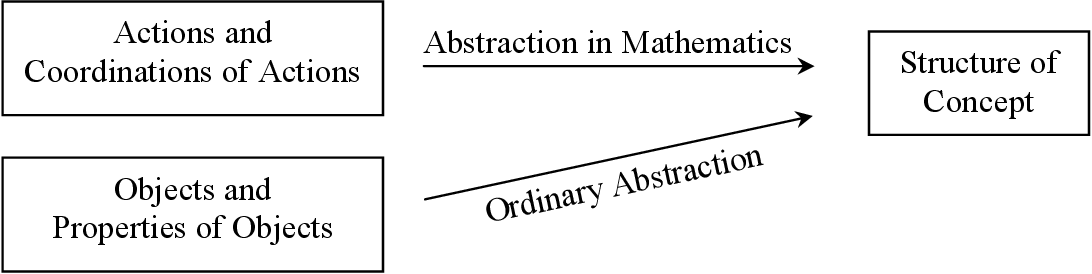•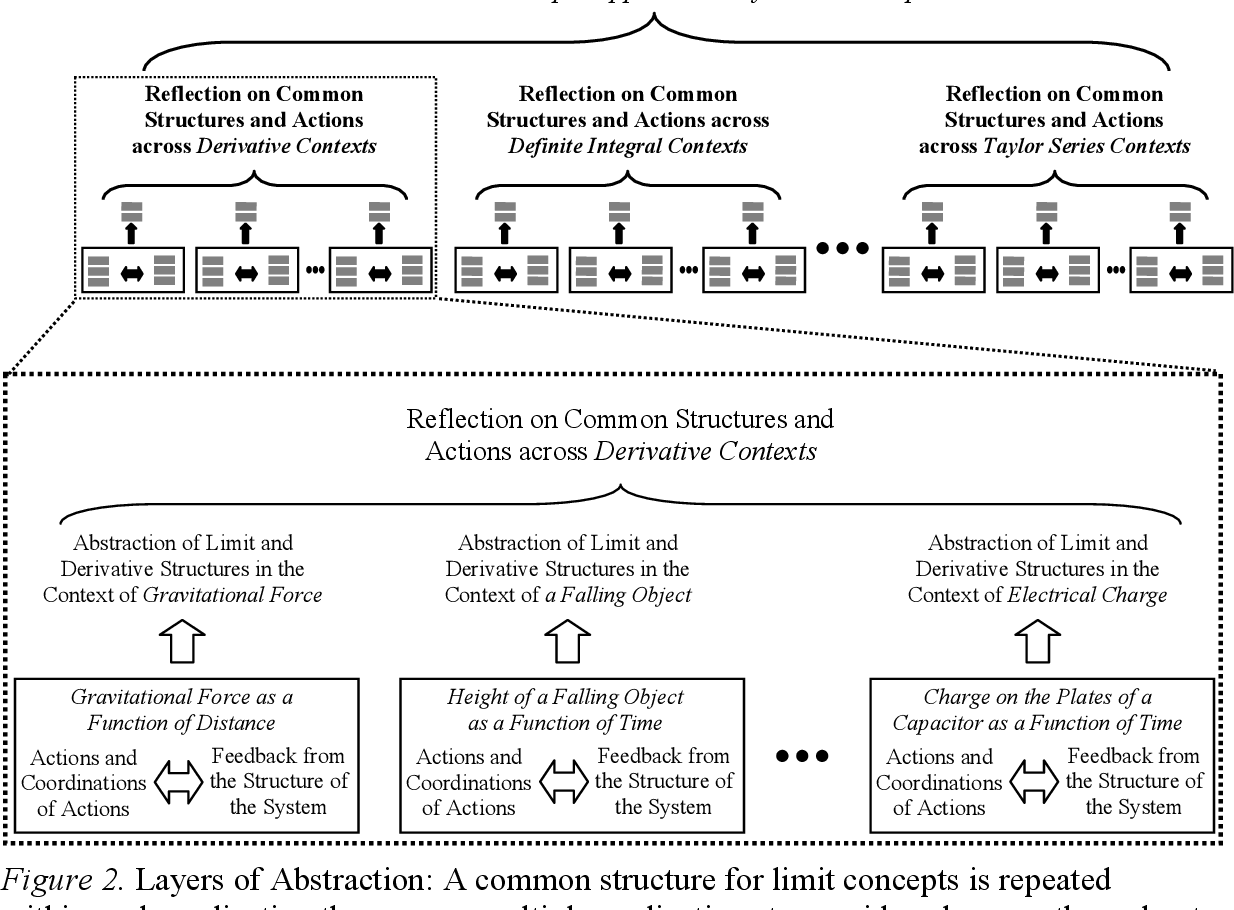•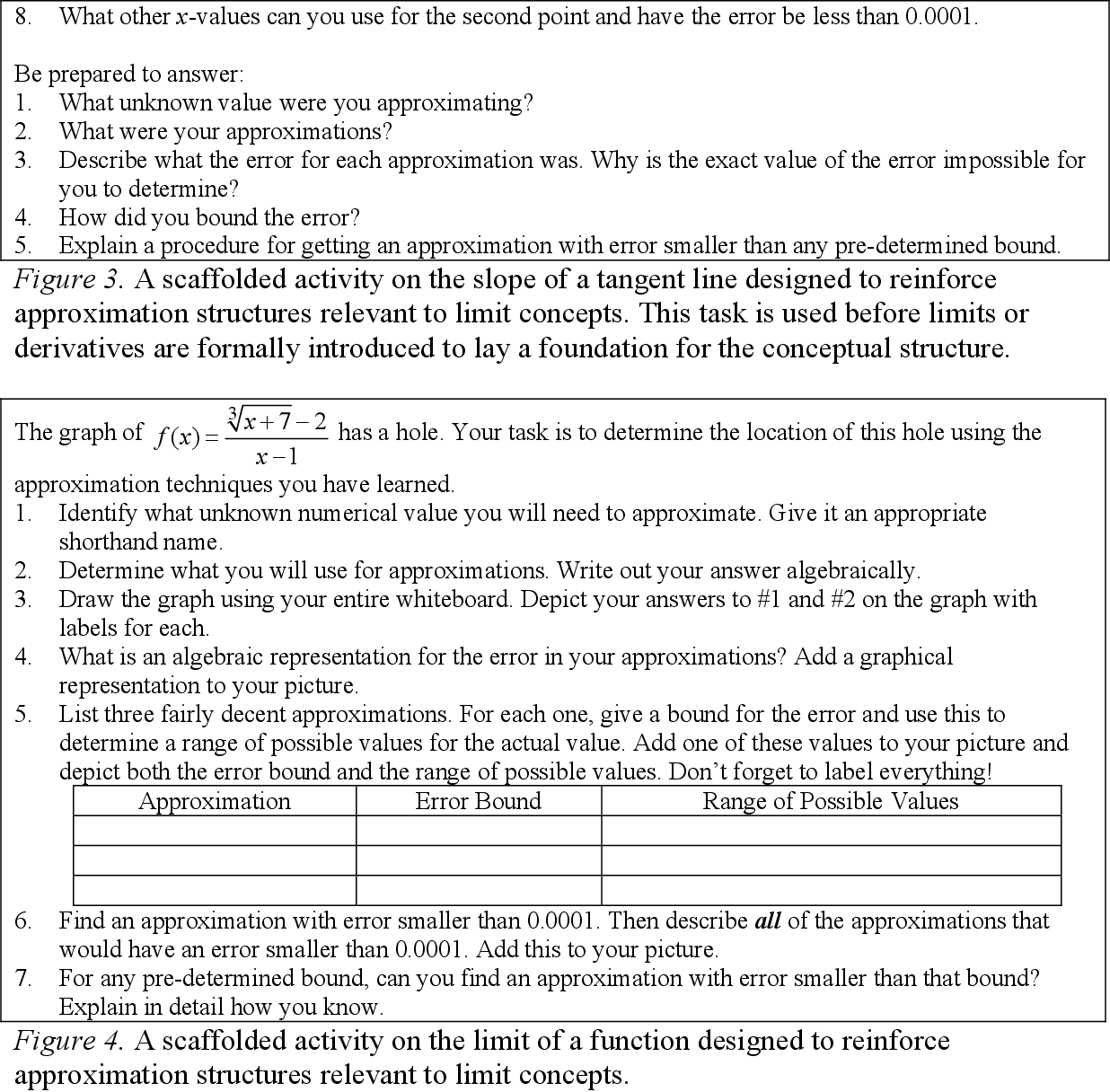•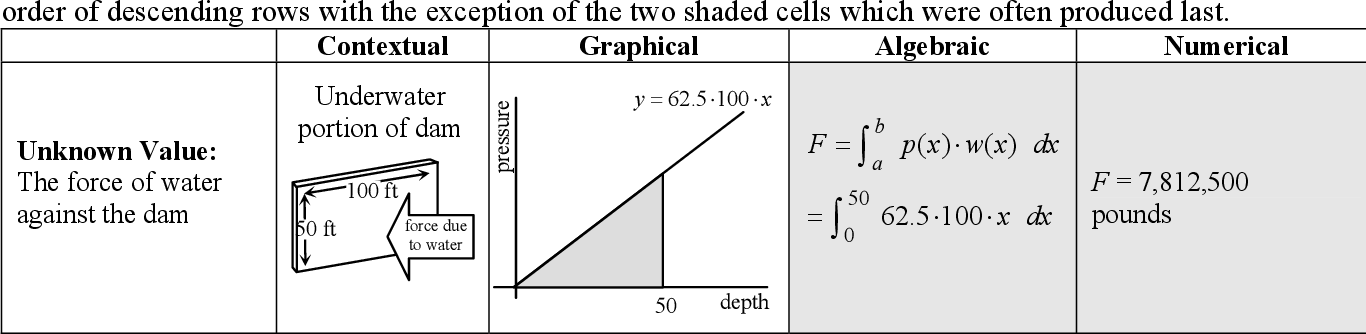Is this relevant?
2004
2004
• SPIE Medical Imaging
• 2004
• Corpus ID: 53135780
Functional magnetic resonance imaging (fMRI) is a technique that is sensitive to correlates of neuronal activity. The application… Expand
Is this relevant?
1998
1998
As part of a project on automatic generation of proofs involving both logic and computation, we have automated the production of… Expand
Is this relevant?
1992
1992
• James F. Blinn
• IEEE Computer Graphics and Applications
• 1992
• Corpus ID: 13495205
The Feynman diagrams, which represent the product of tensors in diagram form as directed graphs, are described. In the diagrams… Expand
Is this relevant?
Highly Cited
1986
Highly Cited
1986
Abstract The epsilon-delta definition of continuity has a natural analog for functions that take lattice points into lattice… Expand
Is this relevant?